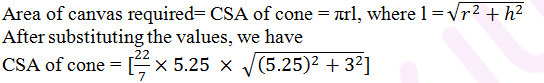Guru

# A heap of wheat is in the form of a cone whose diameter is 10.5 m and height is 3 m. Find its volume. The heap is to be covered by canvas to protect it from rain. Find the area of the canvas. (Assume π = 22/7) Q.9

• 0

what is the tricky way for solving the question of class 9th ncert math of Surface areas and Volumes chapter of ncert of exercise 13.7  of math give me the best and simple way for solving this question in easy and simple way of question no.9 A heap of wheat is in the form of a cone whose diameter is 10.5 m and height is 3 m. Find its volume. The heap is to be covered by canvas to protect it from rain. Find the area of the canvas. (Assume π = 22/7)

Share

1. Radius (r) of heap = (10.5/2) m = 5.25

Height (h) of heap = 3m

Volume of heap = (1/3)πr2h

= (1/3)×(22/7)×5.25×5.25×3

= 86.625

The volume of the heap of wheat is 86.625 m3.

Again,= (22/7)×5.25×6.05

= 99.825

Therefore, the area of the canvas is 99.825 m2.

• 0